# Is This Loss? Part 2: An object recognition model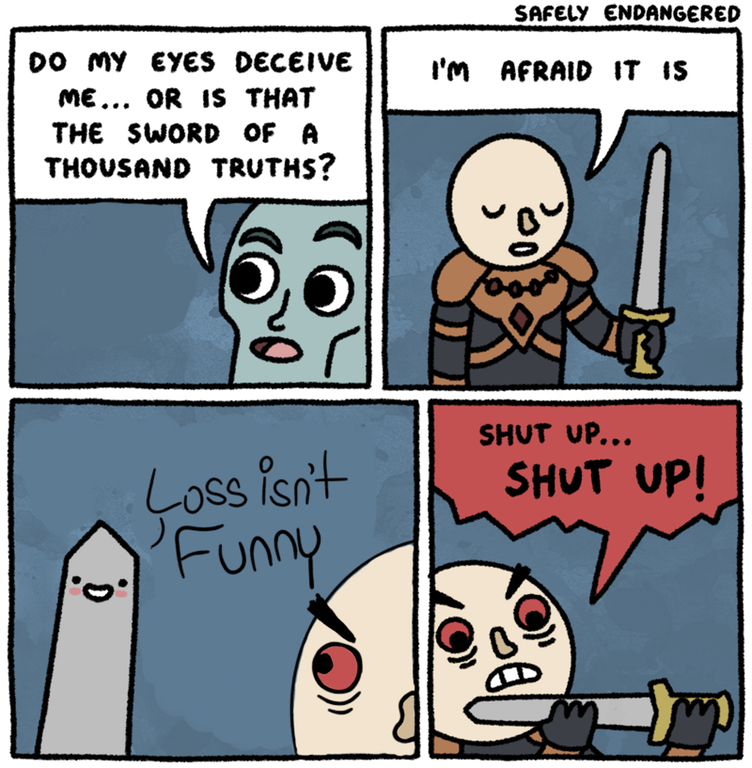/r/me_irl.Original webcomic by Safely Endangered.So in this part I’ll be looking into TensorFlow object detection models and integrate one with the chat bot in part 1.

# Picking A Model

The TensorFlow object detection repository contains some links to pre-trained object detection models, which I’m going to use. I’ve picked `ssd_mobilenet_v1_coco` in this part for its performance, but for training I plan to use faster RCNN because of its higher accuracy.

# ⌥⌘I The Model

To better understand the next step, I’m going to take a look into the model structure and things that we can do with it. Extracting the archive will yield `frozen_inference_graph.pb` among other files. I’m going to use TensorBoard to visualize the inference graph, so I’ll have to convert the inference graph into logs. The TensorFlow repository provides a tool for just that.

Save the script in the extracted directory and run the following command to start TensorBoard.

``````\$ python3 import_pb_to_tensorboard.py --model_dir=frozen_inference_graph.pb --log_dir=logs/
\$ tensorboard --logdir=logs/
TensorBoard 0.1.5 at http://tensorbox.local:6006 (Press CTRL+C to quit)
``````

Now I can inspect the graph with a browser, everything will be wrapped in the `import` namespace, double clicking a namespace reveals what’s inside.

Expand the feature extractor namespace and you can see that Mobilenet is being used as a feature extractor which from the name makes sense. What I need to know to use this model are the op nodes, `image_tensor`, `detection_boxes`, `detection_scores`, and `num_detections`. Those are the outputs while `image_tensor` is the placeholder for the input.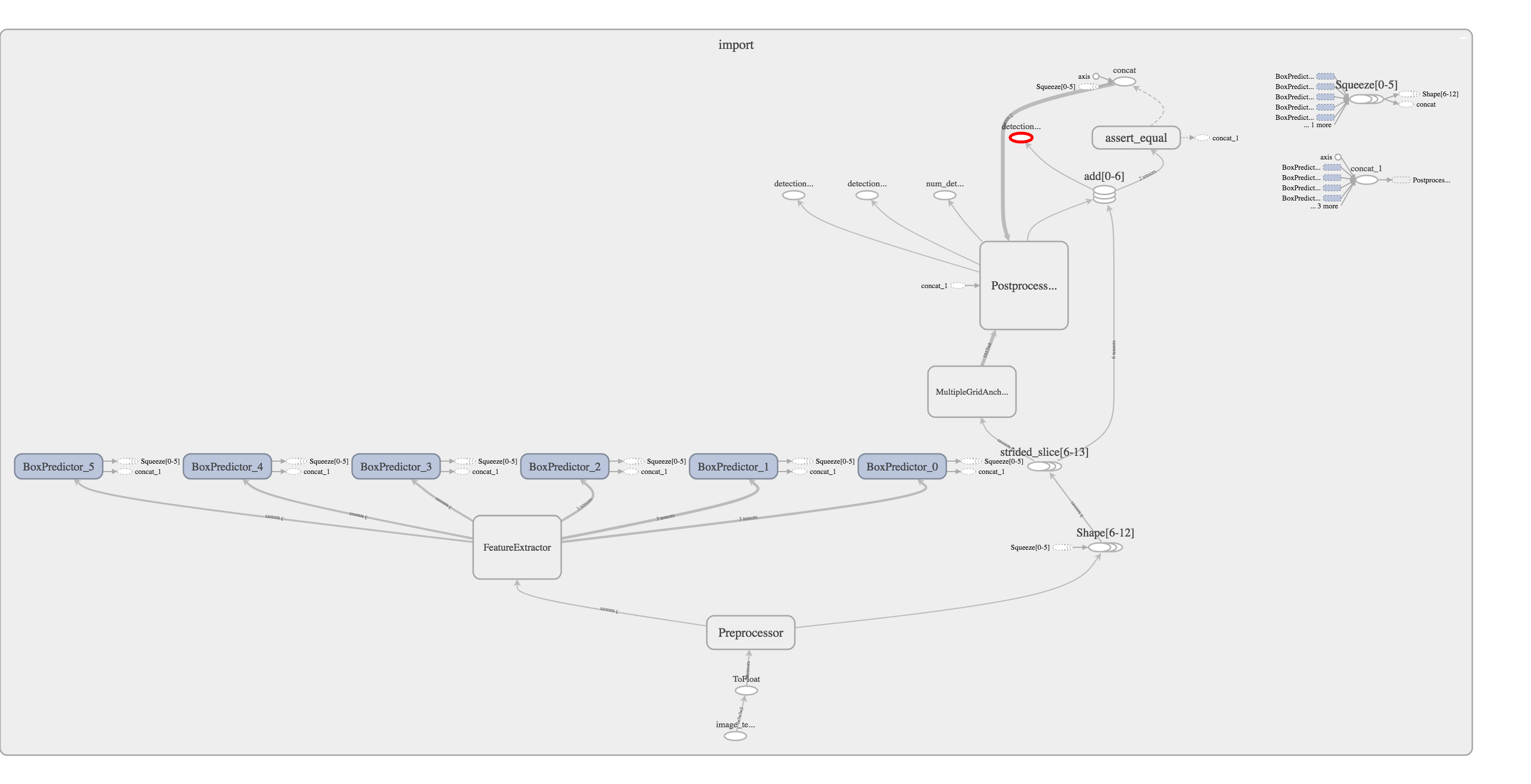Graph of ssd_mobilenet_v1_cocoimage_tensor is at the bottom while the rest are near the top past the post processor.

# Coding the bot

Now I’ll append the code to infer bounding boxes on the images sent, which needs a few more modules. To tap into the plugin life cycle I will override the `load` method to load the model graph into the class’ `graph` attribute.

``````from PIL import ImageDraw
import tensorflow as tf
import numpy as np

self.generate_tensor_dict()

# Loads the file specified by `model_path` into self.graph
self.graph = tf.Graph()
with self.graph.as_default():
# Creates a graph def instance
# This is a representation of the graph definitions
graph_def = tf.GraphDef()

# `GFile` is similar to `open` but more versatile
with tf.gfile.GFile(model_path, 'rb') as f:
# Reads the serialized bytes from the file
# Parse the file as a graph def
# Then imports it into the detection graph
graph_def.ParseFromString(serialized_graph)
# name='' prevents the creation a new `import` namespace
tf.import_graph_def(graph_def, name='')
``````

It will need to load the TensorFlow graph and create a dictionary of tensors intended for output. Recall the op nodes from the previous section, those are the tensors I want.

``````def generate_tensor_dict(self, tensors=[
'num_detections',
'detection_boxes',
'detection_scores',
'detection_classes'
]):
'''
Reads all the tensors into a dictionary like so
{
'tensor_name': <tensor object>
}
'''
self.tensor_dict = {}
with self.graph.as_default():
graph = tf.get_default_graph()
ops = graph.get_operations()
all_tensor_names = {output.name for op in ops for output in op.outputs}

# Iterates through the tensors list and looks for the tensor object
for key in tensors:
# For some reason the tensors have `:0` appending to their names
tensor_name = key + ':0'
if tensor_name in all_tensor_names:
self.tensor_dict[key] = graph.get_tensor_by_name(tensor_name)
``````

The command handler needs to preprocess the image, run the inference, and draw bounding boxes on regions of interest (ROI), before sending it as an attachment.

``````@Plugin.command('!loss', '<link:str...>')
try:
except OSError as e:
event.msg.reply("I can't find a JPEG or PNG image at the link you've given")
return

# Run inference for the image
inference = self.run_inference(image_numpy)
image = self.draw_bounding_boxes(image, inference)

# Send the image with bounding boxes back
file = self.create_attachment_from_image(image)
filename = 'loss_inference.png'
"Hi I found the following image at the link you've given.",
attachments=[(filename, file)])
``````

The first method creates a numpy array from the image data. `run_inference` creates a session, feeds the numpy array into the detection graph, then returns the tensors. The bounding boxes are returned in a normalized format between 0 and 1 indicating it’s relative position on the image. The score is a number between 0 and 1 that indicates the confidence of the bounding box. The detected classes are represented by numbers which can be mapped to a label, but my final product will only have one class: loss.

``````def load_image_into_numpy_array(self, image):
# Convert PIL image into a numpy array like image
(im_width, im_height) = image.size
return np.array(image.getdata()).reshape((im_height, im_width, 3)).astype(np.uint8)

def run_inference(self, image):
with self.graph.as_default():
with tf.Session() as sess:
# Gets the image tensor object
image_tensor = tf.get_default_graph().get_tensor_by_name('image_tensor:0')
# Do forward pass for the output in tensor dict
output = sess.run(self.tensor_dict, feed_dict={
image_tensor: np.expand_dims(image, 0)
})
return output

def draw_bounding_boxes(
self,
image,
inference,
threshold=0.5
):
# Create a draw interface
draw = ImageDraw.Draw(image)
for box, score in zip(inference['detection_boxes'],
inference['detection_scores']):
# Scale the bounding box coordinates
p1 = tuple(box[:2][::-1] * image.size)
p2 = tuple(box[2:][::-1] * image.size)
if score > threshold:
# Draw a red rectange
draw.rectangle([p1, p2], outline=(255, 0, 0))
del draw
return image
``````

The code will be available on my repository once it’s done. For now you can find it on this gist.

# Testing the object detector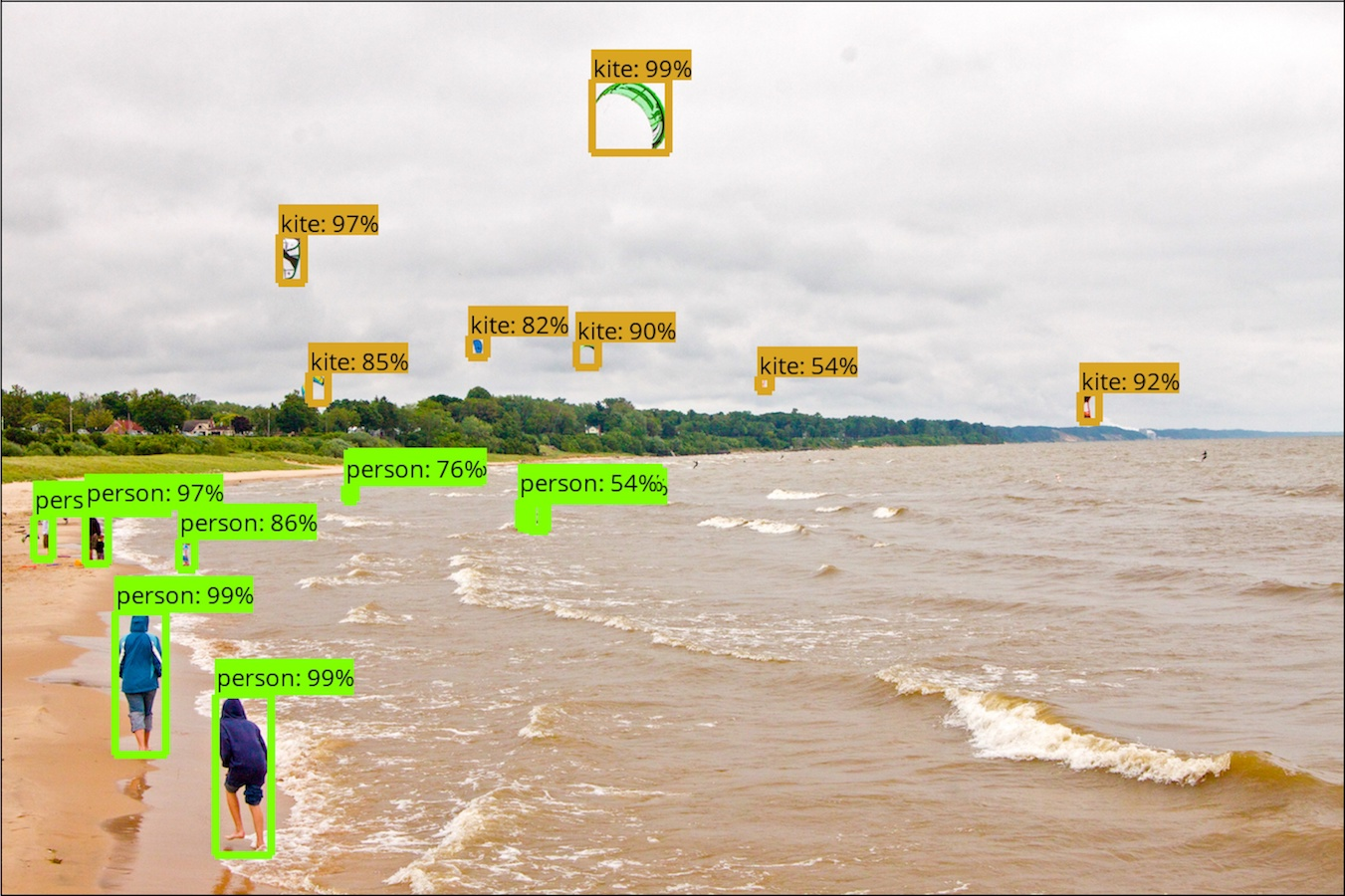Expected outputI’m using this image to test the object detector because I know for sure it works with the current model. Just without the class labels. From TensorFlow object detection API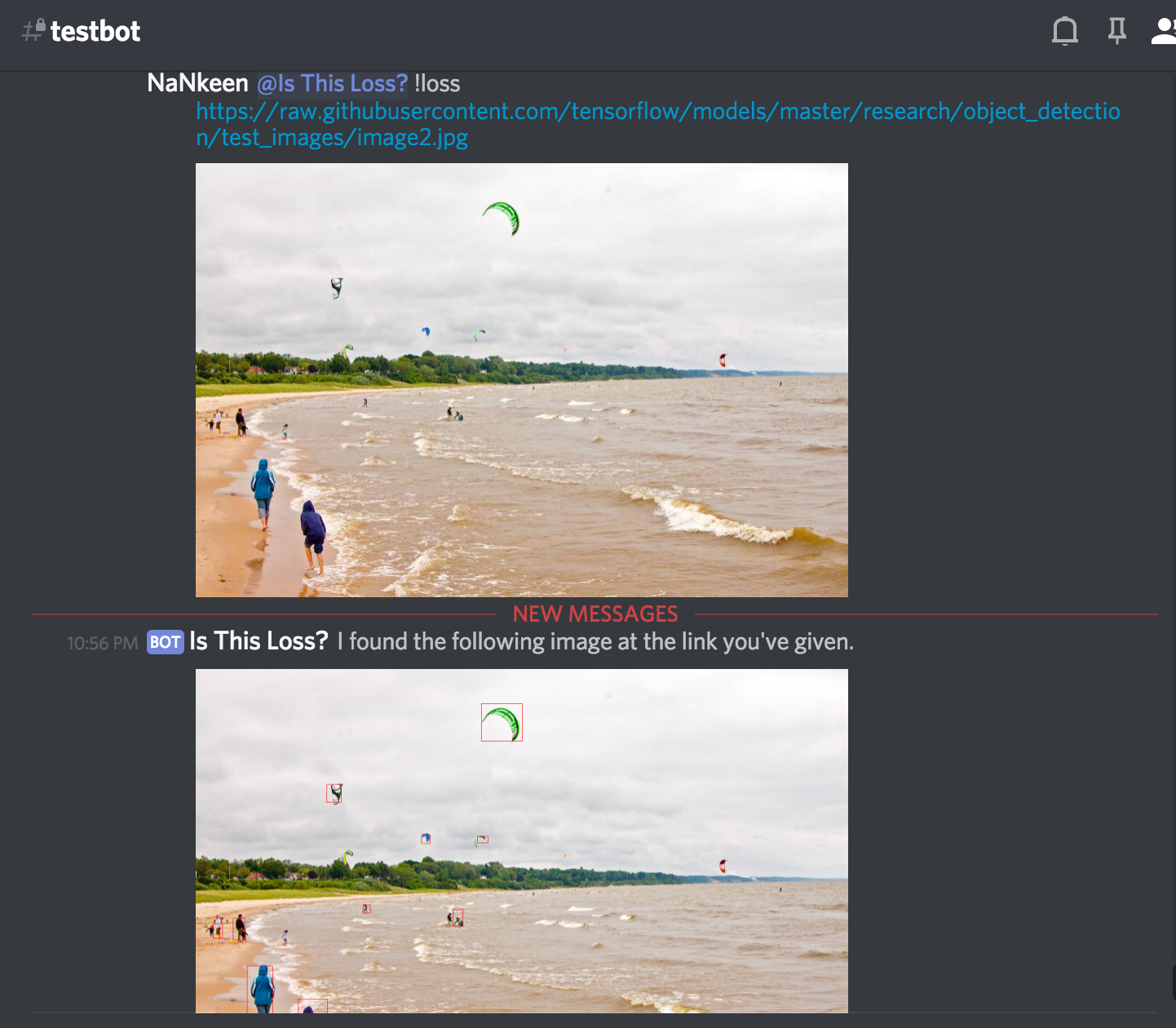In action on DiscordThe lines are thinner as I used a different library to draw the rectangles but if you look closely it’s there.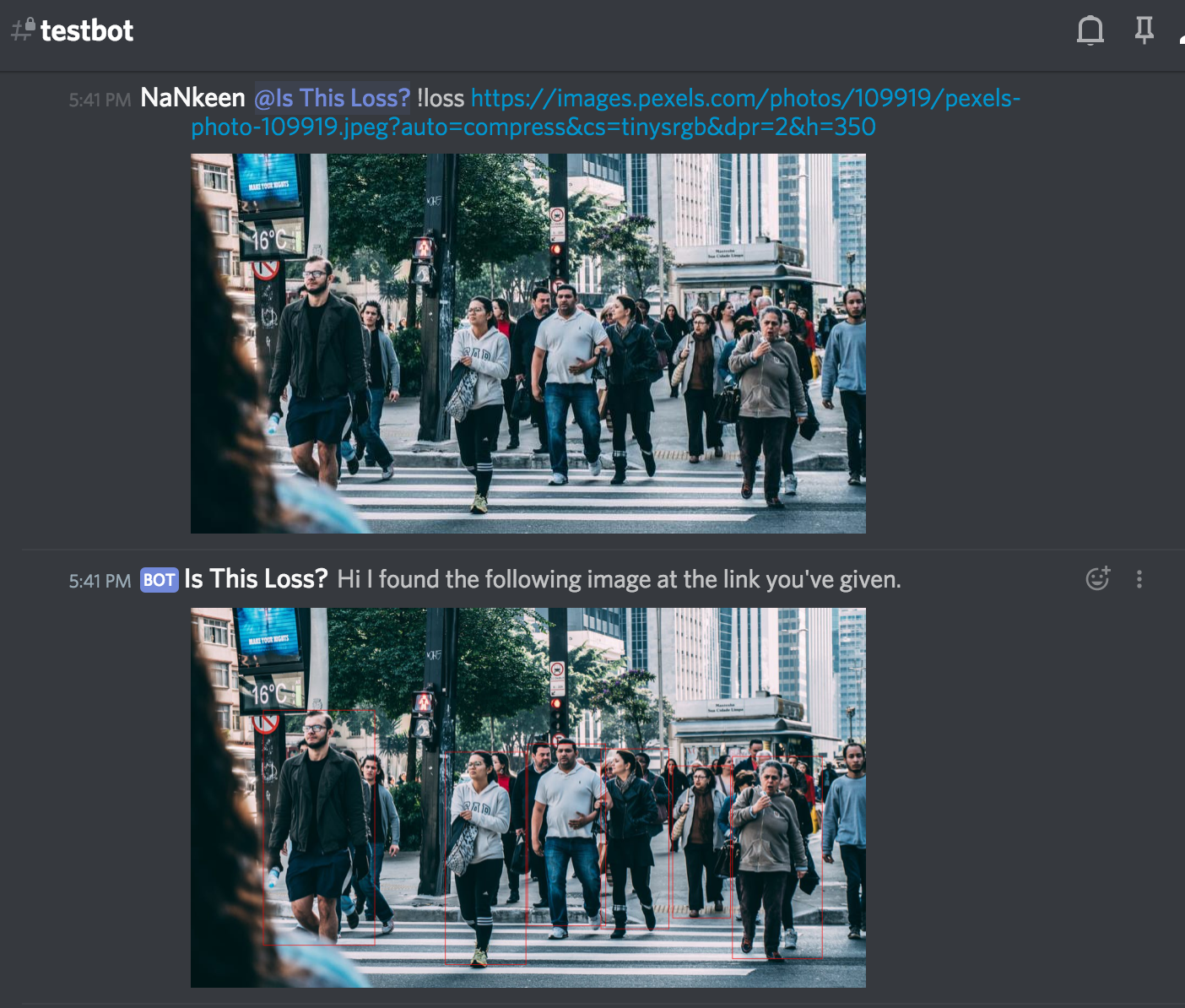Another testThis time it’s on an image I found online, the link is in the picture.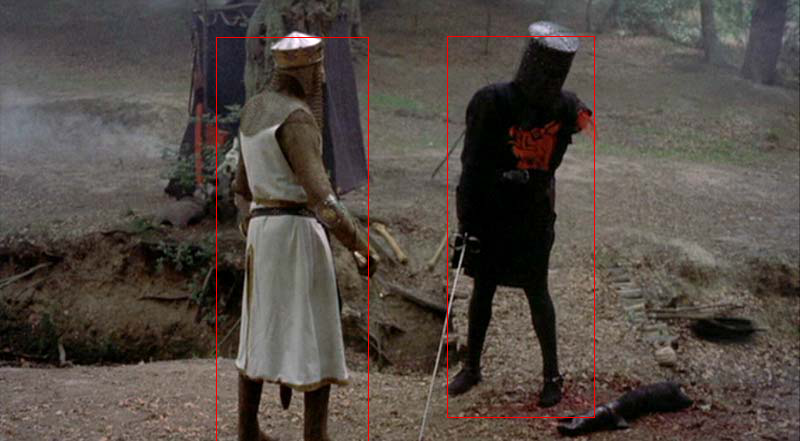Testing on one of my favourite scenes

Object detection has been around for decades and I’m just building with the impressive work of those brilliant individuals who made these models. In the next part I will create the dataset and train one to find loss memes.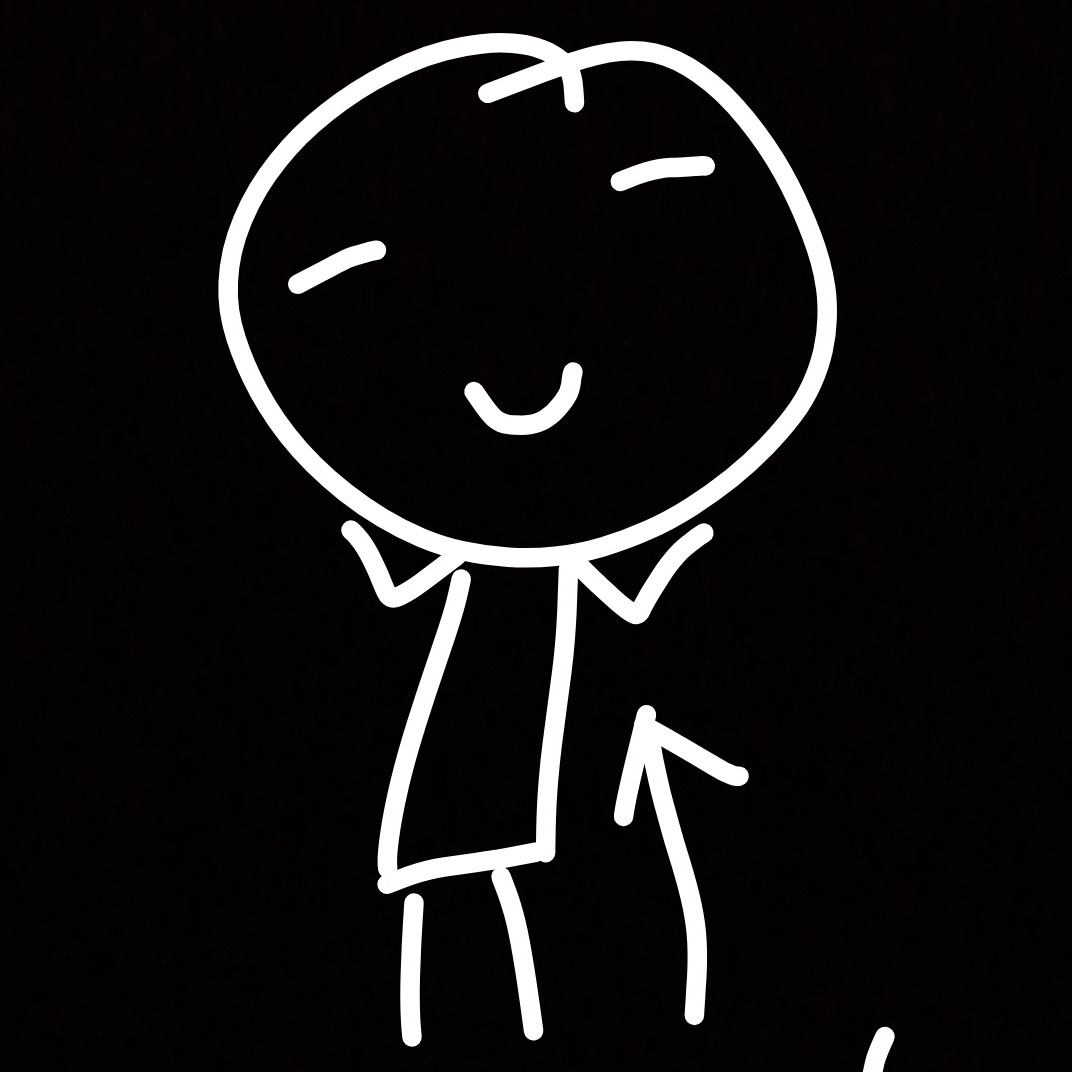# nankeen

Pwn, rev, and stuff.

By Kai, 2018-06-17